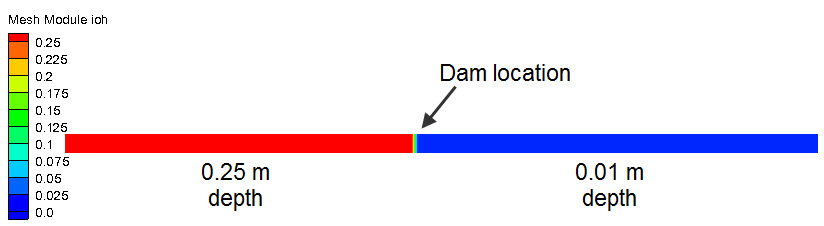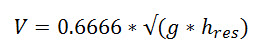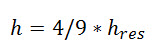## Dam Break

Reference

• Stoker J. J. Water Waves: The Mathematical Theory with Applications. Institute of Mathematical Sciences. New York University, New York. 1957. page 337.

Domain

• rectangular, flat-bottomed flume
• 7.3 m length
• 0.18 m width
• Dam at x = 3.37 m

Initial Conditions

• Upstream reservoir depth = 0.25 m
• Downstream reservoir depth = 0.01 mBoundary Conditions

• Dam Break at t = 0 s

Run Files

• 2D-dambreak.3dm
• 2D-dambreak.bc
• 2D-dambreak.hotResults• Velocity

​• Analytic velocity = 1.04 m/s
• Model Velocity = 1.034 m/s
• Depth• Analytic Depth = = 0.111m
• Model Depth = 0.110 to 0.112 m